# Java程序设计基础--------运算符

## 1.算数运算符

Java 语言中的运算符除了具有优先级之外，还有一个结合性的特点。当一个表达式中出现多种运算符时，执行的先后顺序不仅要遵守运算符优先级别的规定，还要受运算符结合性的约束，以便确定是自左向右进行运算还是自右向左进行运算。这些运算符按照操作数的数量可以分为单目运算符、双目运算符和三目运算符。

Java 中的算术运算符主要用来组织数值类型数据的算术运算，按照参加运算的操作数的不同可以分为一元运算符和二元运算符。

### 1.1一元运算符

- 取反符号 取反运算 b=-a
++ 自加一 先取值再加一，或先加一再取值 a++ 或 ++a

-a 是对 a 取反运算，a++ 或 a-- 是在表达式运算完后，再给 a 加一或减一。而 ++a 或 --a 是先给 a 加一或减一，然后再进行表达式运算。

int a = 12;
System.out.println(-a);
int b = a++;
System.out.println(b);
b = ++a;
System.out.println(b);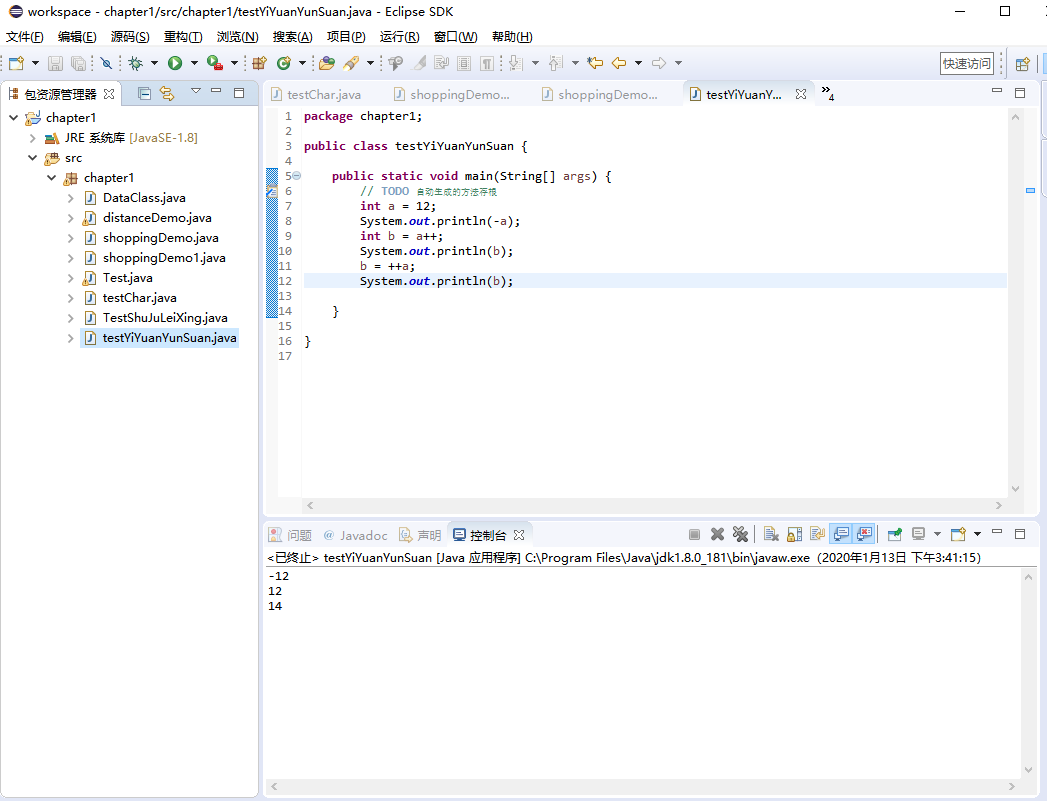### 1.2二元运算符

Java 语言中算术运算符的功能是进行算术运算，除了经常使用的加（+）、减（-）、乘（*）和除（\）外，还有取模运算（％）。加（+）、减（-）、乘（*）、除（\）和我们平常接触的数学运算具有相同的含义。

+ 求 a 加 b 的和，还可用于 String 类型，进行字符串连接操作 a + b
- 求 a 减 b 的差 a - b
* 求 a 乘以 b 的积 a * b
/ 求 a 除以 b 的商 a / b
% 取余 求 a 除以 b 的余数 a % b

int a = 4, b = 2, c = 3;
int d = a * (b + c) % c;


• 求余（％）运算要求参与运算的两个操作数均为整型，不能为其他类型。
• 两个整数进行除法运算，其结果仍为整数。如果整数与实数进行除法运算，则结果为实数。

public static void main(String[] args) {
float f1 = 9 % 4;// 保存取余后浮点类型的结果
double da = 9 + 4.5; // 双精度加法
double db = 9 - 3.0; // 双精度减法
double dc = 9 * 2.5; // 双精度乘法
double dd = 9 / 3.0; // 双精度除法
double de = 9 % 4; // 双精度取余
System.out.println("整数的算术运算"); // 整数的加、减、乘、除和取余
System.out.printf("9+4=%d \n", 9 + 4);
System.out.printf("9-4=%d \n", 9 - 4);
System.out.printf("9*4=%d \n", 9 * 4);
System.out.printf("9/4=%d \n", 9 / 4);
System.out.printf("9%%4=%d \n", 9 % 4);
System.out.println("\n浮点数的算术运算"); // 浮点数的加、减、乘、除和取余
System.out.printf("9+4.5f=%f \n", 9 + 4.5f);
System.out.printf("9-3.0f=%f \n", 9 - 3.0f);
System.out.printf("9*2.5f=%f \n", 9 * 2.5f);
System.out.printf("9/3.0f=%f \n", 9 / 3.0f);
System.out.printf("9%%4=%f \n", f1);
System.out.println("\n双精度数的算术运算"); // 双精度数的加、减、乘、除和取余
System.out.printf("9+4.5=%4.16f \n", da);
System.out.printf("9-3.0=%4.16f \n", db);
System.out.printf("9*2.5=%4.16f \n", dc);
System.out.printf("9/3.0=%4.16f \n", dd);
System.out.printf("9%%4=%4.16f \n", de);
System.out.println("\n字符的算术运算"); // 对字符的加法和减法
System.out.printf("'A'+32=%d \n", 'A' + 32);
System.out.printf("'A'+32=%c \n", 'A' + 32);
System.out.printf("'a'-'B'=%d \n", 'a' - 'B');
}


整数的算术运算
9+4=13
9-4=5
9*4=36
9/4=2
9%4=1

9+4.5f=13.500000
9-3.0f=6.000000
9*2.5f=22.500000
9/3.0f=3.000000
9%4=1.000000

9+4.5=13.5000000000000000
9-3.0=6.0000000000000000
9*2.5=22.5000000000000000
9/3.0=3.0000000000000000
9%4=1.0000000000000000

'A'+32=97
'A'+32=a
'a'-'B'=31


### 1.3算数赋值运算符

+= 加赋值 a += b、a += b+3
-= 减赋值 a -= b
*= 乘赋值 a *= b
/= 除赋值 a /= b
%= 取余赋值 a %= b

int a = 1;
int b = 2;
a += b; // 相当于 a = a + b
System.out.println(a);
a += b + 3; // 相当于 a = a + b + 3
System.out.println(a);
a -= b; // 相当于 a = a - b
System.out.println(a);
a *= b; // 相当于 a=a*b
System.out.println(a);
a /= b; // 相当于 a=a/b
System.out.println(a);
a %= b; // 相当于 a=a%b
System.out.println(a);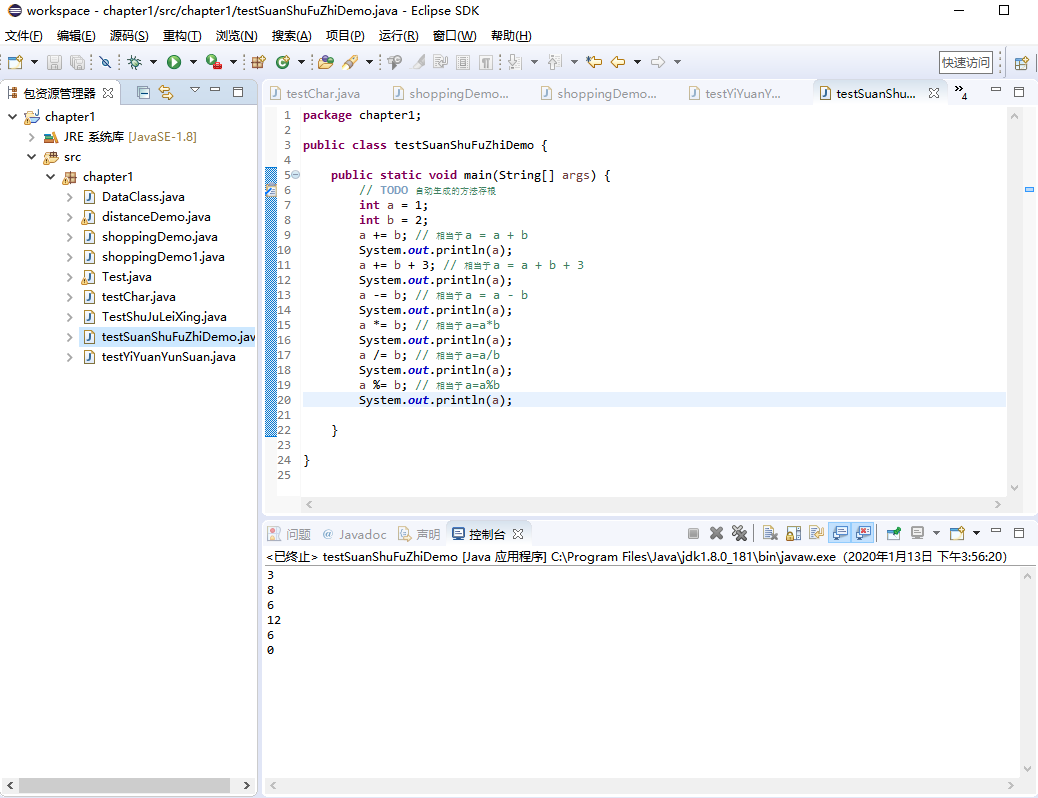## 2.赋值运算符

变量名称=表达式内容


Java 语言中，“变量名称”和“表达式”内容的类型必须匹配，如果类型不匹配则需要自动转化为对应的类型。

+= 将该运算符左边的数值加上右边的数值， 其结果赋值给左边变量本身 int a=5; a+=2; a=7
-= 将该运算符左边的数值减去右边的数值， 其结果赋值给左边变量本身 int a=5; a-=2; a=3
*= 将该运算符左边的数值乘以右边的数值， 其结果赋值给左边变量本身 int a=5; a*=2; a=10
/= 将该运算符左边的数值整除右边的数值， 其结果赋值给左边变量本身 int a=5; a/=2; a=2
%= 将该运算符左边的数值除以右边的数值后取余，其结果赋值给左边变量本身 int a=5; a%=2; a=1

int x, y, z; // 定义3个整型的变量
x = y = z = 5; // 为变量赋初值为5
x += 10; // 等价于x=x+10，结果x=15
y -= 3; // 等价于y=y-3，结果y=2
z *= 5; // 等价于z=z*5，结果z=25
x /= 4; // 等价于x=x/4，结果x=3
z %= x; // 等价于z=z%x，结果z=1


public static void main(String[] args) {
double price = 10.25; // 定义商品的单价，赋值为10.25
double total = 0; // 定义总价初始为0
int count = 2; // 定义购买数量，赋值为2
price -= 1.25; // 减去降价得到当前单价
count *= 5; // 现在需要购买10个，即原来数量的5倍
total = price * count; // 总价=当前单价*数量
System.out.printf("商品当前的单价为：%4.2f \n", price); // 输出当前单价
System.out.printf("购买商品的数量为：%d \n", count); // 输出购买数量
System.out.printf("总价为：%4.2f \n", total); // 输出总价
}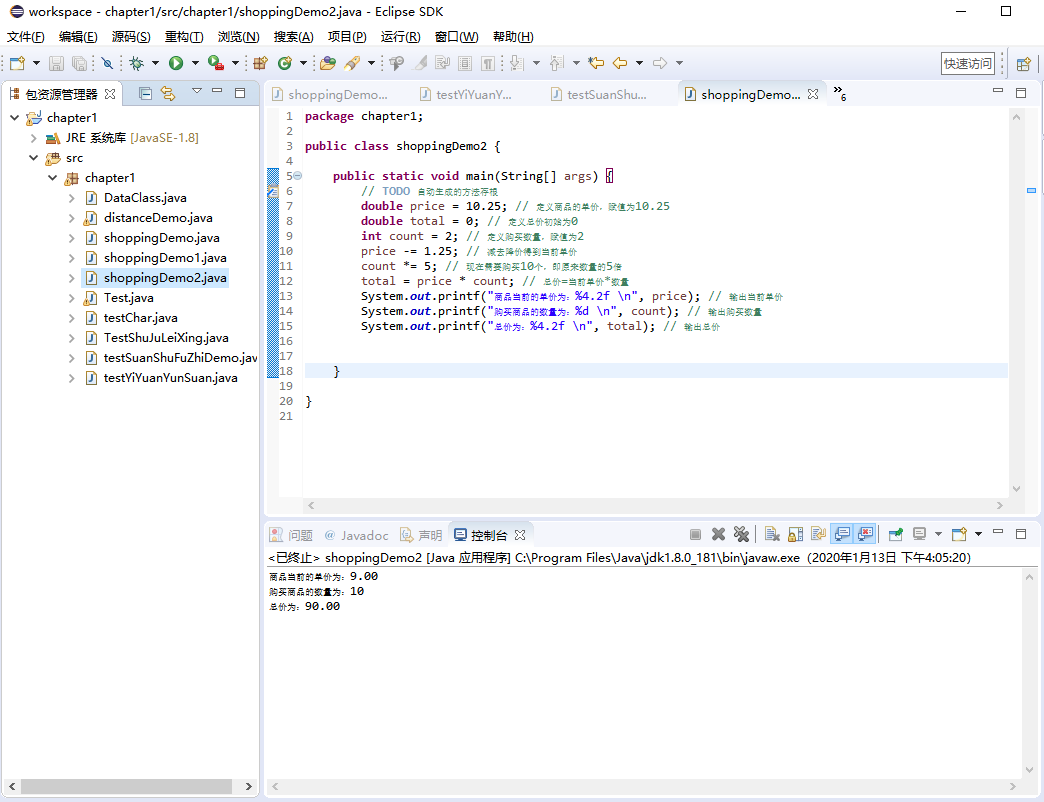double d1 = 12.34
double d2 = d1 + 5; // 将表达式的值赋给d2
System.out.println(d2); // 输出 d2 的值，将输出 17.34


## 3.逻辑运算符

&& a&&b 短路与 ab 全为 true 时，计算结果为 true，否则为 false。 2>1&&3<4 true
|| a||b 短路或 ab 全为 false 时，计算结果为 false，否则为 true。 2<1||3>4 flase
!a 逻辑非 a 为 true 时，值为 false，a 为 false 时，值为 true !(2>4) true
| a|b 逻辑或 ab 全为 false 时，计算结果为 false，否则为 true 1>2|3>5 flase
& a&b 逻辑与 ab 全为 false 时，计算结果为 false，否则为 true 1<2&3<5 true
• && 与 & 区别：如果 a 为 false，则不计算 b（因为不论 b 为何值，结果都为 false）
• || 与 | 区别：如果 a 为 true，则不计算 b（因为不论 b 为何值，结果都为 true）

a b a&&b a||b !a
true true true true flase
flase true flase true true
true flase flase true flase
flase flase flase flase true

x>0 && x<=100    // 第一行语句
y%4==0 || y%3==0    // 第二行语句
!(x>y)    // 第三行语句


## 4.关系运算符

> 大于运算符 只支持左右两边操作数是数值类型。如果前面变量的值大于后面变量的值， 则返回 true。 2>3 flase
>= 大于或等于运算符 只支持左右两边操作数是数值类型。如果前面变量的值大于等于后面变量的值， 则返回 true。 4>=2 true
< 小于运算符 只支持左右两边操作数是数值类型。如果前面变量的值小于后面变量的值，则返回 true。 2<3 true
<= 小于或等于运算符 只支持左右两边操作数是数值类型。如果前面变量的值小于等于后面变量的值， 则返回 true。 4<=2 flase
== 相等运算符 如果进行比较的两个操作数都是数值类型，无论它们的数据类型是否相同，只要它们的值不相等，也都将返回 true。

Java 也支持两个 boolean 类型的值进行比较。
44
97
’a’
5.05
true
false
true
true
true
flase
!= 不相等运算符 如果进行比较的两个操作数都是数值类型，无论它们的数据类型是否相同，只要它们的值不相等，也都将返回 true。

4!=2 true

1. 基本类型的变量、值不能和引用类型的变量、值使用 == 进行比较；boolean 类型的变量、值不能与其他任意类型的变量、值使用 == 进行比较；如果两个引用类型之间没有父子继承关系，那么它们的变量也不能使用 == 进行比较。
2. == 和 != 可以应用于基本数据类型和引用类型。当用于引用类型比较时，比较的是两个引用是否指向同一个对象，但当时实际开发过程多数情况下，只是比较对象的内容是否相当，不需要比较是否为同一个对象。

• 运算符 >=、==、！=、<= 是两个字符构成的一个运算符，用空格从中分开写就会产生语法错误。例如 x> =y;是错误的，但是可以写成x >= y; 在运算符的两侧增加空格会提高可读性。同样将运算符写反，例如 =>、=<、=! 等形式会产生语法错误。
• 由于计算机内存放的实数与实际的实数存在着一定的误差，如果对浮点数进行 ==（相等）或 !=（不相等）的比较，容易产生错误结果，应该尽量避免。
• 不要将“==”写成“=”。

a > b    // 比较变量a的值是否大于变量b的值
x+y> = z    // 比较变量x与变量y的和是否大于或等于变量z的值
width * width+size != area    // 比较变量width的平方加上变量size的值是否与变量area的值不相等
name == "zhht"    // 比较变量name的值是否等于字符串nzht
pass != "123456"    // 比较变量pass的值是否不等于字符串“123456”


import java.util.Scanner;
public static void main(String[] args) {
int number1, number2; // 定义变量，保存输入的两个数
System.out.print("请输入第一个整数(number1)：");
Scanner input = new Scanner(System.in);
number1 = input.nextInt(); // 输入第一个数
System.out.print("请输入第二个整数(number2)：");
input = new Scanner(System.in);
number2 = input.nextInt(); // 输入第二个数
System.out.printf("number1=%d,number2=%d\n", number1, number2); // 输出这两个数
// 判断用户输入的两个数是否相等
if (number1 == number2) {
System.out.println("number1 和 number2 相等。");
}
// 判断用户输入的两个数据是否相等
if (number1 != number2) {
System.out.println("number1 和 number2 不相等。");
// 判断用户输入的数1是否大于数2
if (number1 > number2) {
System.out.println("number1 大于 number2。");
}
// 判断用户输入的数1是否小于数2
if (number1 < number2) {
System.out.println("number1 小于 number2。");
}
}
}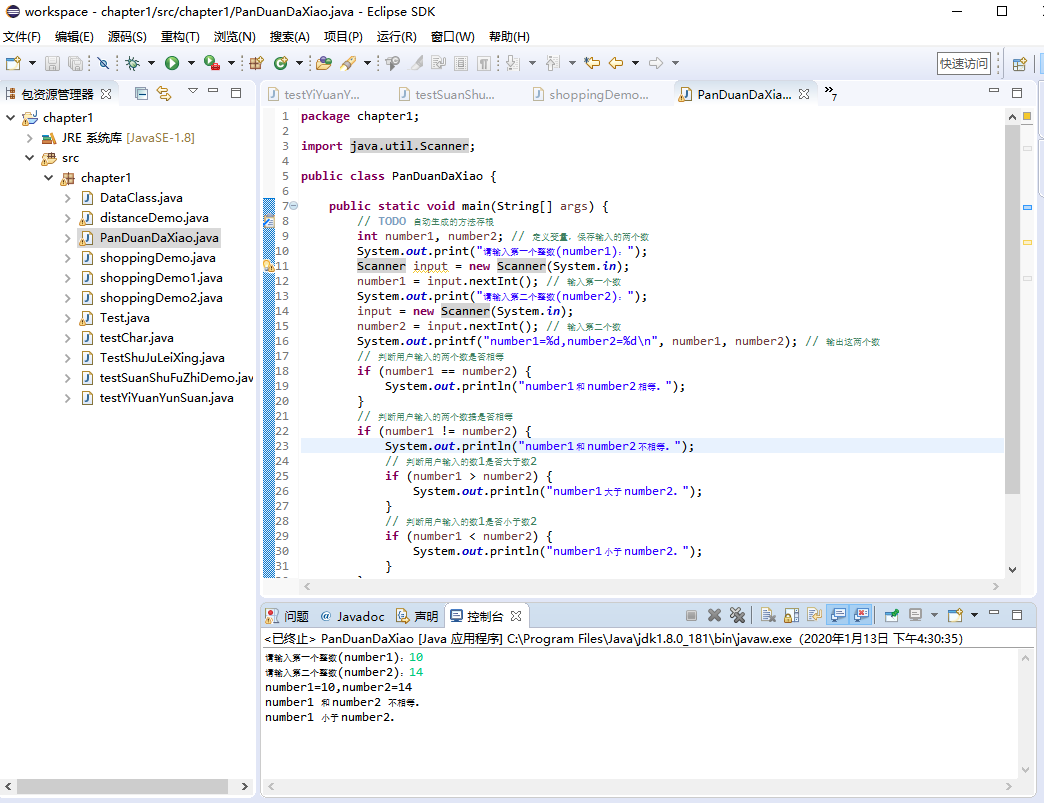## 5.自增自减运算符

i++ 将 i 的值先使用再加 1 赋值给 i 变量本身 int i=1;
int j=i++;
i=2
j=1
++i 将 i 的值先加 1 赋值给变量 i 本身后再使用 int i=1;
int j=++i;
i=2
j=2
i– 将 i 的值先使用再减 1 赋值给变量 i 本身 int i=1;
int j=i–;
i=0
j=1
–i 将 i 的值先减 1 后赋值给变量 i 本身再使用 int i=1;
int j=–i;
i=0
j=0

• 自增/自减只能作用于变量，不允许对常量、表达式或其他类型的变量进行操作。常见的错误是试图将自增或自减运算符用于非简单变量表达式中。
• 自增/自减运算可以用于整数类型 byte、short、int、long，浮点类型 float、double，以及字符串类型 char。
• Java 1.5 以上版本中，自增/自减运算可以用于基本类型对应的包装器类 Byte、Short、Integer、Long、Float、Double 和 Character。
• 自增/自减运算结果的类型与被运算的变量类型相同。

public static void main(String[] args) {
int x = 5, y; // 声明用于自增和自减的整型变量
char cx = 'B', cy; // 声明用于自增和自减的字符型变量
float fx = 5.5f, fy; // 声明用于自增和自减的浮点型变量
System.out.println("---------对整数的自增和自减---------");
y = x++;
System.out.printf("y=x++ 的结果为：%d ,%d \n", x, y);
y = x--;
System.out.printf("y=x-- 的结果为：%d ,%d \n", x, y);
y = ++x;
System.out.printf("y=++x 的结果为：%d ,%d \n", x, y);
y = --x;
System.out.printf("y=--x 的结果为：%d ,%d \n", x, y);
System.out.println("\n---------对浮点的自增和自减---------");
fy = fx++;
System.out.printf("fy=fx++ 的结果为：%f ,%f \n", fx, fy);
fy = fx--;
System.out.printf("fy=fx-- 的结果为：%f ,%f \n", fx, fy);
fy = ++fx;
System.out.printf("fy=++fx 的结果为：%f ,%f \n", fx, fy);
fy = --fx;
System.out.printf("fy=--fx 的结果为：%f ,%f \n", fx, fy);
System.out.println("\n---------对字符的自增和自减---------");
cy = cx++;
System.out.printf("cy=cx++ 的结果为：%c ,%c \n", cx, cy);
cy = cx--;
System.out.printf("cy=cx-- 的结果为：%c ,%c \n", cx, cy);
cy = ++cx;
System.out.printf("cy=++cx 的结果为：%c ,%c \n", cx, cy);
cy = --cx;
System.out.printf("cy=--cx 的结果为：%c ,%c \n", cx, cy);
}


---------对整数的自增和自减---------
y=x++ 的结果为：6 ,5
y=x-- 的结果为：5 ,6
y=++x 的结果为：6 ,6
y=--x 的结果为：5 ,5

---------对浮点的自增和自减---------
fy=fx++ 的结果为：6.500000 ,5.500000
fy=fx-- 的结果为：5.500000 ,6.500000
fy=++fx 的结果为：6.500000 ,6.500000
fy=--fx 的结果为：5.500000 ,5.500000

---------对字符的自增和自减---------
cy=cx++ 的结果为：C ,B
cy=cx-- 的结果为：B ,C
cy=++cx 的结果为：C ,C
cy=--cx 的结果为：B ,B


## 6.位运算符

Java 定义的位运算（bitwise operators）直接对整数类型的位进行操作，这些整数类型包括 long，int，short，char 和 byte。

Java 语言中的位运算符分为位逻辑运算符和位移运算符两类，下面详细介绍每类包含的运算符。

### 6.1位逻辑运算符

& 按位进行与运算（AND） 4 & 5 4
| 按位进行或运算（OR） 4|5 5
^ 按位进行异或运算（XOR） 4 ^ 5 1
~ 按位进行取反运算（NOT） ~ 4 -5

### 6.2位与运算符

100&0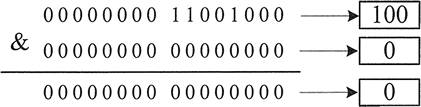int x = 5,y = 12; // 创建整型变量保存两个数
int z = x&y; // 对这两个数进行位与运算，结果保存到z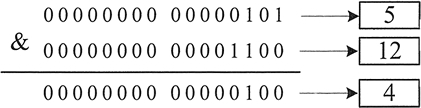### 6.3位或运算符

11|7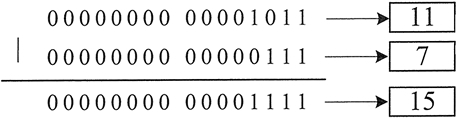### 6.4位异或运算符

11^7


### 6.5位取反运算符

~10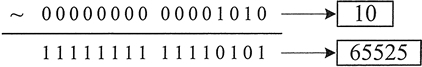int i = 10;
System.out.printf("%d \n",~i);


int i=10;
System.out.printf("%x \n",~i);


### 6.6位移运算符

>> 右移位运算符 8>>1 4
<< 左移位运算符 9<<2 36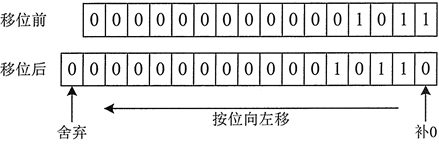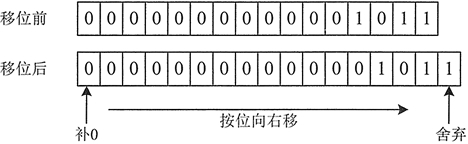### 6.7复合位赋值运算

&= 按位与赋值 num1 &= num2 等价于 num 1=num 1 & num2
|= 按位或赋值 num1 |= num2 等价于 num 1=num 1 | num2
^= 按位异或赋值 num1 ^= num2 等价于 num 1=num 1 ^ num2
-= 按位取反赋值 num1 ~= num2 等价于 num 1=num 1 ~ num2
<<= 按位左移赋值 num1 «= num2 等价于 num 1=num 1 « num2
>>= 按位右移赋值 num1 »= num2 等价于 num 1=num 1 » num2

int a = 1;
int b = 2;
int c = 3;
a &= 4;
a |= 4;
a ^= c;
a -= 6;
b >>= 1;
c <<= 1;
System.out.println("a = " + a);
System.out.println("b = " + b);
System.out.println("c = " + c);


a = 1
b = 1
c = 6


## 7.条件运算符

Java 提供了一个特别的三元运算符（也叫三目运算符）经常用于取代某个类型的 if-then-else 语句。条件运算符的符号表示为“?:”，使用该运算符时需要有三个操作数，因此称其为三目运算符。使用条件运算符的一般语法结构为：

result = <expression> ? <statement1> : <statement3>;


int x,y,z;
x = 6,y = 2;
z = x>y ? x-y : x+y;


x>y ? x-=y : x+=y;


(x>y ? x-=y : x)+=y;


(x>y) ? (x-=y): (x+=y);


import java.util.Scanner;
public class Test9 {
public static void main(String[] args) {
int x, y, z; // 声明三个变量
System.out.print("请输入一个数：");
Scanner input = new Scanner(System.in);
x = input.nextInt(); // 由用户输入x的值
// 判断x的值是否大于5，如果是y=x，否则y=-x
y = x > 5 ? x : -x;
// 判断y的值是否大于x，如果是z=y，否则z=5
z = y > x ? y : 5;
System.out.printf("x=%d \n", x);
System.out.printf("y=%d \n", y);
System.out.printf("z=%d \n", z);
}
}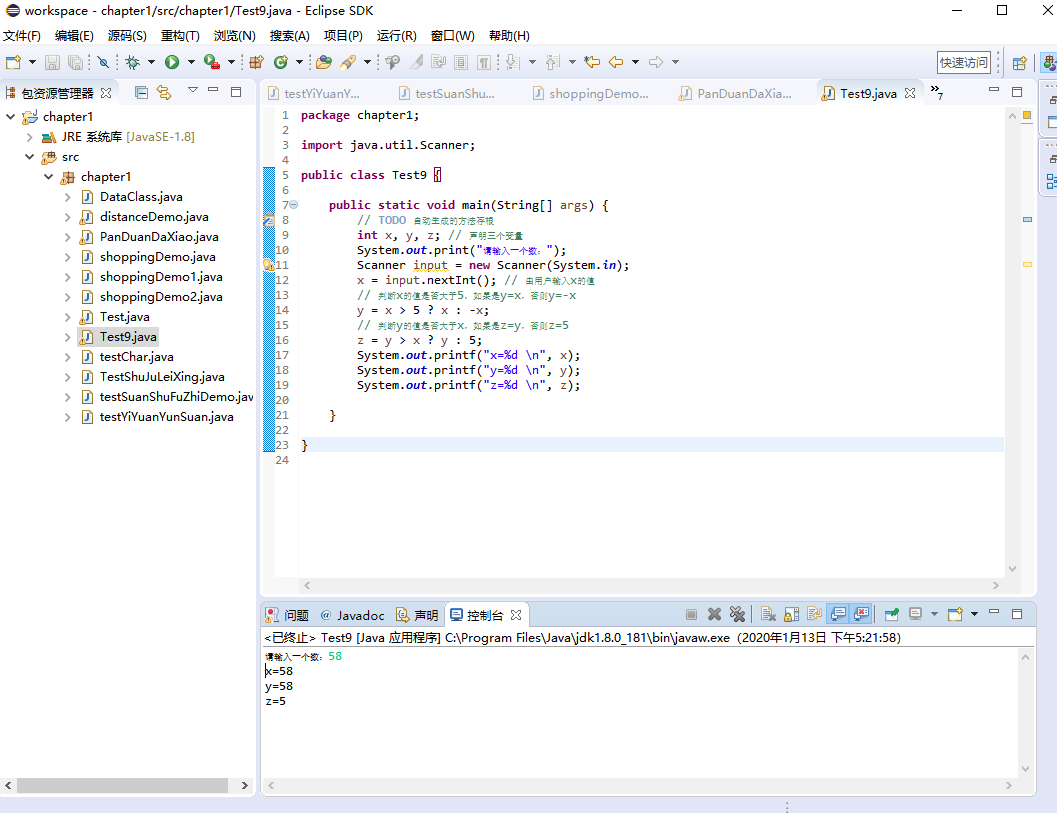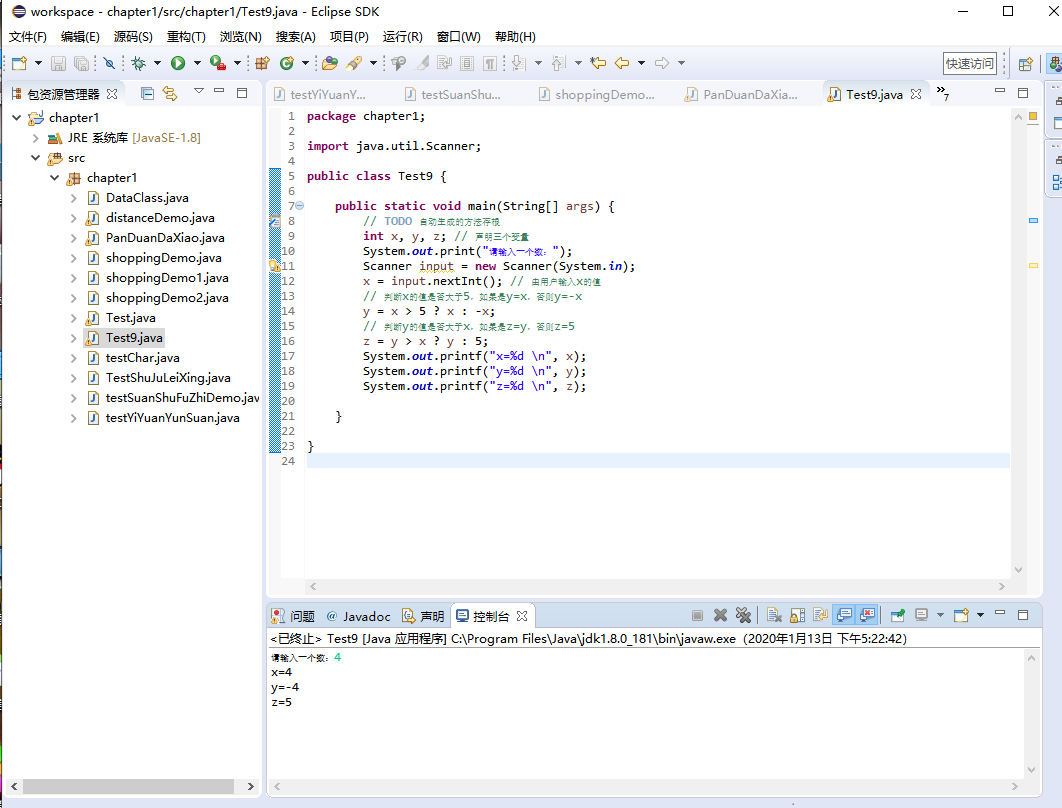## 8.运算符优先级

Java 语言中运算符的优先级共分为 14 级，其中 1 级最高，14 级最低。在同一个表达式中运算符优先级高的先执行。

1 （）、[]、{} 从左向右
2 !、+、-、~、++、– 从右向左
3 *、/、% 从左向右
4 +、- 从左向右
5 «、»、>>> 从左向右
6 <、<=、>、>=、instanceof 从左向右
7 ==、!= 从左向右
8 & 从左向右
9 ^ 从左向右
10 | 从左向右
11 && 从左向右
12 || 从左向右
13 ?: 从右向左
14 =、+=、-=、*=、/=、&=、|=、^=、~=、«=、»=、>>>= 从右向左

(x-y)*z/5


--y || ++x && ++z;


① 先计算 y 的自减运算符，即 --y。
② 再计算 x 的自增运算符，即 ++x。
③ 接着计算 z 的自增运算符，即 ++z。
④ 由于逻辑与比逻辑或的优先级高，这里将 ② 和 ③ 的结果进行逻辑与运算，即 ++x && ++z。
⑤ 最后将 ④ 的结果与 ① 进行逻辑或运算，即 --y||++x&&++z。

(--y) || ((++x)&&(++z));


int a = 5;
int b = 4;
int c = a++- --b*++a/b-- >>2%a--;


• 不要把一个表达式写得过于复杂，如果一个表达式过于复杂，则把它分成几步来完成。
• 不要过多地依赖运算符的优先级来控制表达式的执行顺序，这样可读性太差，尽量使用()来控制表达式的执行顺序。

## 9.直接量

### 9.1直接量的类型

#### 6）char 类型的直接量

char 类型的直接量有三种形式，分别是用单引号括起来的字符、转义字符和 Unicode 值表示的字符。例如‘a’，‘\n’和‘\u0061’。

### 9.2直接量的赋值

int a = 5;
char c = 'a';
boolean b = true;
float f = 5.12f;
double d = 4.12;
String name = "C语言中文网";
String url = "http://c.biancheng.net";


String 类型的直接量不能赋给其他类型的变量，null 类型的直接量可以直接赋给任何引用类型的变量，包括 String 类型。boolean 类型的直接量只能赋给 boolean 类型的变量，不能赋给其他任何类型的变量。

• 由于 String 类是一个典型的不可变类，因此 String 对象创建出来的就不可能改变，因此无需担心共享 String 对象会导致混乱。
• 常量池（constant pool）指的是在编译期被确定，并被保存在已编译的 .class 文件中的一些数据，它包括关于类、方法、接口中的常量，也包括字符串直接量。

String s0 = "hello";
String s1 = "hello";
String s2 = "he" + "llo";
System.out.println(s0 == s1);
System.out.println(s0 == s2);


true
true


String 类型的直接量不能赋给其他类型的变量，null 类型的直接量可以直接赋给任何引用类型的变量，包括 String 类型。boolean 类型的直接量只能赋给 boolean 类型的变量，不能赋给其他任何类型的变量。

• 由于 String 类是一个典型的不可变类，因此 String 对象创建出来的就不可能改变，因此无需担心共享 String 对象会导致混乱。
• 常量池（constant pool）指的是在编译期被确定，并被保存在已编译的 .class 文件中的一些数据，它包括关于类、方法、接口中的常量，也包括字符串直接量。

String s0 = "hello";
String s1 = "hello";
String s2 = "he" + "llo";
System.out.println(s0 == s1);
System.out.println(s0 == s2);


true
true


Java 会确保每个字符串常量只有一个，不会产生多个副本。例子中的 s0 和 s1 中的“hello”都是字符串常量，它们在编译期就被确定了，所以 s0 = s1 返回 true。而“he”和“llo”也都是字符串常量，当一个字符串由多个字符串常量连接而成时，它本身也是字符串常量，s2 同样在编译期就被解析为一个字符串常量，所以 s2 也是常量池中“hello”的引用。因此，程序输出 s0 == s1 返回 true，s1 == s2 也返回 true。©️2019 CSDN 皮肤主题: 黑客帝国 设计师: 上身试试